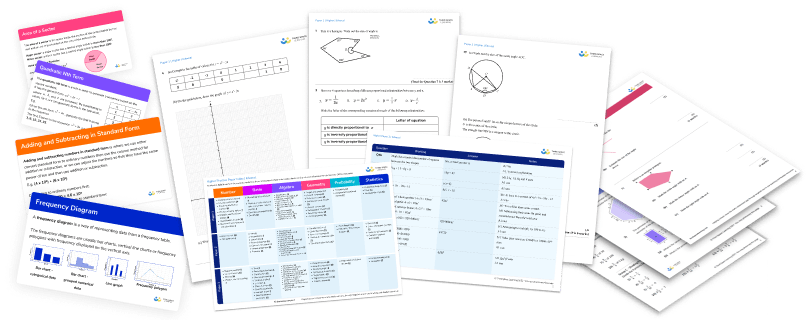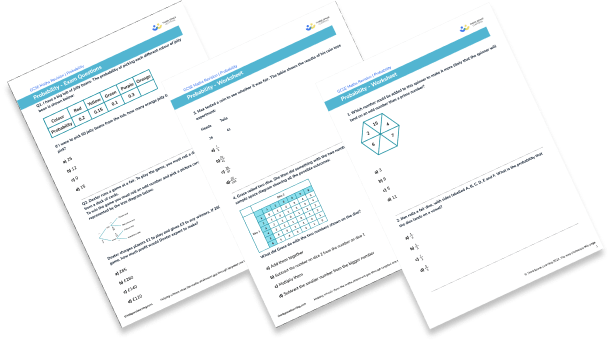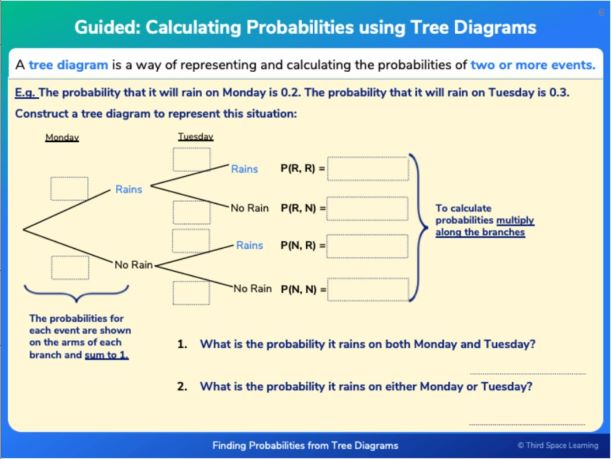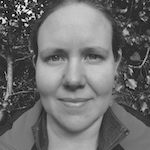Teaching support from the UK’s largest provider of in-school maths tuition.one to one lessonsschools supported

Shape confident mathematicians

One to one tuition designed to build confidence and engagement in an interactive low-stakes environment

See it in action

FREE secondary maths resources

Here you can find all of our free secondary resources; explore the collections or navigate to each section to filter resources by topicExplore all resources
Secondary Worksheets & Practice Questions

Shape confident mathematicians

One to one tuition designed to build confidence and engagement in an interactive low-stakes environment

See it in action

# 15 Probability Questions And Practice Problems (KS3 & KS4): Harder GCSE Exam Style Questions Included

Probability questions and probability problems require students to work out how likely it is that something is to happen. Probabilities can be described using words or numbers. Probabilities range from 0 to 1 and can be written as fractions, decimals or percentages.

Here you’ll find a selection of probability questions of varying difficulty showing the variety you are likely to encounter in KS3 and KS4 including several GCSE exam style questions.

Free GCSE maths revision resources for schools
As part of the Third Space Learning offer to schools, the personalised online GCSE maths tuition can be supplemented by hundreds of free GCSE maths revision resources including:
GCSE maths past papers
GCSE maths worksheets
GCSE maths questions

### What are some real life examples of probability?

The more likely something is to happen, the higher its probability. We think about probabilities all the time. For example, you may have seen that there is a 20% chance of rain on a certain day or thought about how likely you are to roll a 6 when playing a game, or to win in a raffle when you buy a ticket.

### How to calculate probabilities

The probability of something happening is given by:

$\text{Probability} = \frac{\text{the number of ways an event can occur}}{\text{the total number of possible outcomes}}$

We can also use the following formulae to help us calculate probabilities and solve problems:

• Probability of something not occuring = 1 – probability of if occurring

P(not\;A) = 1 - P(A)

• For mutually exclusive events:

Probability of event A OR event B occurring = Probability of event A +
Probability of event B

P(A\;or\;B) = P(A)+P(B)

• For independent events:

Probability of event A AND event B occurring = Probability of event A times probability of event B

P(A\;and\;B) = P(A) × P(B)Download this 15 Probability Questions And Practice Problems (KS3 & KS4) WorksheetDownload Free Now!

### KS3 probability questions

In KS3 probability questions introduce the idea of the probability scale and the fact that probabilities sum to one. We look at theoretical and experimental probability as well as learning about sample space diagrams and venn diagrams.

#### Year 7 probability questions

1. Which number could be added to this spinner to make it more likely that the spinner will land on an odd number than a prime number?39511Currently there are two odd numbers and two prime numbers so the chances of landing on an odd number or a prime number are the same. By adding 3, 5 or 11 you would be adding one prime number and one odd number so the chances would remain equal.

By adding 9 you would be adding an odd number but not a prime number. There would be three odd numbers and two prime numbers so the spinner would be more likely to land on an odd number than a prime number.

2. Ifan rolls a fair dice, with sides labelled A, B, C, D, E and F. What is the probability that the dice lands on a vowel?

\frac{1}{6}\frac{1}{3}\frac{1}{2}\frac{2}{3}A and E are vowels so there are 2 outcomes that are vowels out of 6 outcomes altogether.

Therefore the probability is   \frac{2}{6} which can be simplified to \frac{1}{3} .

#### Year 8 probability questions

3. Max tested a coin to see whether it was fair. The table shows the results of his coin toss experiment:

26                  41

What is the relative frequency of the coin landing on heads?

\frac{1}{2}\frac{26}{41}\frac{26}{67}\frac{26}{100}Max tossed the coin 67 times and it landed on heads 26 times.

\text{Relative frequency (experimental probability) } = \frac{\text{number of successful trials}}{\text{total number of trials}} = \frac{26}{67}

4. Grace rolled two dice. She then did something with the two numbers shown. Here is a sample space diagram showing all the possible outcomes:What did Grace do with the two numbers shown on the dice?Subtract the number on dice 2 from the number on dice 1Multiply themSubtract the smaller number from the bigger numberFor each pair of numbers, Grace subtracted the smaller number from the bigger number.

For example, if she rolled a 2 and a 5, she did 5 − 2 = 3.

#### Year 9 probability questions

5. Alice has some red balls and some blue balls in a bag. Altogether she has 25 balls. Alice picks one ball from the bag. The probability that Alice picks a red ball is x and the probability that Alice picks a blue ball is 4x. Work out how many blue balls are in the bag.

6100205Since the probability of mutually exclusive events add to 1:

\begin{aligned} x+4x&=1\\\\ 5x&=1\\\\ x&=\frac{1}{5} \end{aligned}

\frac{1}{5} of the balls are red and \frac{4}{5} of the balls are blue.

\frac{4}{5} \text{  of  } 25 = 20

6. Arthur asked the students in his class whether they like maths and whether they like science. He recorded his results in the venn diagram below.How many students don’t like science?

162376We need to look at the numbers that are not in the ‘Like science’ circle. In this case it is 9 + 7 = 16.

### KS4 probability questions

In KS4 probability questions involve more problem solving to make predictions about the probability of an event. We also learn about probability tree diagrams, which can be used to represent multiple events, and conditional probability.One of the first probability slides on tree diagrams for GCSE students on Third Space Learning’s online intervention.

#### Year 10 probability questions

7. A restaurant offers the following options:

Main – chicken, fish or vegetarian

Dessert – ice cream or cake

How many possible different combinations of starter, main and dessert are there?

712827The number of different combinations is 2 × 3 × 2 = 12.

8. There are 18 girls and 12 boys in a class. \frac{2}{9} of the girls and \frac{1}{4} of the boys walk to school. One of the students who walks to school is chosen at random. Find the probability that the student is a boy.

\frac{12}{30}\frac{3}{7}\frac{1}{4}\frac{3}{12}First we need to work out how many students walk to school:

\frac{2}{9} \text{ of } 18 = 4

\frac{1}{4} \text{ of } 12 = 3

4 + 3 = 7

7 students walk to school. 4 are girls and 3 are boys. So the probability the student is a boy is \frac{3}{7} .

9. Rachel flips a biased coin. The probability that she gets two heads is 0.16. What is the probability that she gets two tails?

0.840.60.360.7056We have been given the probability of getting two heads. We need to calculate the probability of getting a head on each flip.

Let’s call the probability of getting a head p.

The probability p, of getting a head AND getting another head is 0.16.

Therefore to find p:

\begin{aligned} p × p = 0.16\\\\ p^2 = 0.16\\\\ p = 0.4. \end{aligned}

The probability of getting a head is 0.4 so the probability of getting a tail is 0.6.

The probability of getting two tails is 0.6 × 0.6 = 0.36.

#### GCSE foundation probability questions

10. I have a big tub of jelly beans. The probability of picking each different colour of jelly bean is shown below:If I were to pick 60 jelly beans from the tub, how many orange jelly beans would I expect to pick?

2512015First we need to calculate the probability of picking an orange. Probabilities sum to 1 so 1 − (0.2 + 0.15 + 0.1 + 0.3) = 0.25.

The probability of picking an orange is 0.25.

The number of times I would expect to pick an orange jelly bean is 0.25 × 60 = 15.

11. Dexter runs a game at a fair. To play the game, you must roll a dice and pick a card from a deck of cards.

To win the game you must roll an odd number and pick a picture card. The game can be represented by the tree diagram below.Dexter charges players £1 to play and gives £3 to any winners. If 260 people play the game, how much profit would Dexter expect to make?

£65£260£140£120Completing the tree diagram:Probability of winning is \frac{1}{2} \times \frac{4}{13} = \frac{4}{26}

If 260 play the game, Dexter would receive £260.

The expected number of winners would be \frac{4}{26} \times 260 = 40

Dexter would need to give away 40 × £3 = £120.

Therefore Dexter’s profit would be £260 − £120 = £140.

12. A coin is tossed three times. Work out the probability of getting two heads and one tail.

\frac{1}{8}\frac{3}{8}\frac{1}{2}\frac{1}{6}There are three ways of getting two heads and one tail: HHT, HTH or THH.

The probability of each is \frac{1}{2} \times \frac{1}{2} \times \frac{1}{2} = \frac{1}{8}

Therefore the total probability is \frac{1}{8} +\frac{1}{8} + \frac{1}{8} = \frac{3}{8}

#### GCSE higher probability questions

13. 200 people were asked about which athletics event they thought was the most exciting to watch. The results are shown in the table below.A person is chosen at random. Given that that person chose 100m, what is the probability that the person was female?

\frac{32}{200}\frac{32}{100}\frac{32}{88}\frac{32}{56}Since we know that the person chose 100m, we need to include the people in that column only.

In total 88 people chose 100m so the probability the person was female is \frac{32}{88}  .

14.  Sam asked 50 people whether they like vegetable pizza or pepperoni pizza.

37 people like vegetable pizza.

25 people like both.

3 people like neither.

Sam picked one of the 50 people at random. Given that the person he chose likes pepperoni pizza, find the probability that they don’t like vegetable pizza.

\frac{12}{50}\frac{3}{50}\frac{12}{35}\frac{10}{35}We need to draw a venn diagram to work this out.

We start by putting the 25 who like both in the middle section. The 37 people who like vegetable pizza includes the 25 who like both, so 12 more people must like vegetable pizza. 3 don’t like either. We have 50 – 12 – 25 – 3 = 10 people left so this is the number that must like only pepperoni.There are 35 people altogether who like pepperoni pizza. Of these, 10 do not like vegetable pizza. The probability is   \frac{10}{35} .

15. There are 12 marbles in a bag. There are n red marbles and the rest are blue marbles. Nico takes 2 marbles from the bag. Write an expression involving n for the probability that Nico takes one red marble and one blue marble.

\frac{n(12-n)}{66}\frac{n(n-1)}{132}\frac{(12-n)(11-n)}{132}\frac{n(12-n)}{132}We need to think about this using a tree diagram. If there are 12 marbles altogether and n are red then 12-n are blue.To get one red and one blue, Nico could choose red then blue or blue then red so the probability is:

\begin{aligned} \frac{n}{12} \times \frac{12-n}{11} + \frac{12-n}{11} \times \frac{n}{11} &= \frac{n(12-n)}{132} + \frac{n(12-n)}{132}\\\\ &= \frac{2n(12-n)}{132}\\\\ &=\frac{n(12-n)}{66} \end{aligned}

#### Looking for more probability questions and resources?

Third Space Learning’s free GCSE maths resource library contains detailed lessons with step-by-step instructions on how to solve ratio problems, as well as worksheets with ratio and proportion practice questions and more GCSE exam questions.

Take a look at the probability lessons today – more are added every week.

#### Looking for more KS3 and KS4 maths questions?

Try these:

Do you have students who need extra support in maths?
Every week Third Space Learning’s maths specialist tutors support thousands of students across hundreds of schools with weekly online 1-to-1 lessons and maths interventions designed to plug gaps and boost progress.

Since 2013 we’ve helped over 150,000 primary and secondary students become more confident, able mathematicians. Find out more about our GCSE Maths tuition or request a personalised quote for your school to speak to us about your school’s needs and how we can help.

Secondary school tuition designed to plug gaps and prepare KS3 and KS4 students for the challenges ahead.##### Beki Christian
Maths Teacher and Author
Beki has 11 years experience teaching secondary Maths. She is currently working as a Maths tutor as well as writing resources and blog posts for Third Space Learning.

Related Articles

x#### Download this 15 Probability Questions And Practice Problems (KS3 & KS4) Worksheet